• matlab开发-活动轮廓简化测试平台。几种主动轮廓分割方法的实现与演示。
• 无边界活动轮廓模型matlab源码，亲自测试，保证有效。
• 设v(s)=[x(s),y(s)]为活动轮廓线，s∈[0, 1]是弧长，其能量函数为： 曲线能量的第一项是曲线的一阶导和二阶导，为曲线的内部能量，其中一阶导为连续能量，控制连续；二阶导到为曲率能量，控制平...

1. Snake模型
人为地在图像感兴趣的区域（ROI）上给出初始轮廓曲线，最小化一个能量函数，使轮廓曲线在图像中运动（变形），最终逼近该区域的边界。
设v(s)=[x(s),y(s)]为活动轮廓线，s∈[0, 1]是弧长，其能量函数为：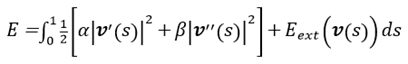曲线能量的第一项是曲线的一阶导和二阶导，为曲线的内部能量，其中一阶导为连续能量，控制连续；二阶导到为曲率能量，控制平滑。能量的第二项是外部能量，一般为下面的形式：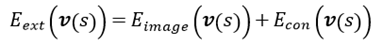其中Eimage为轮廓与图像特征之间的吻合程度，如Eimage(v(s))=±[▽Gσ(x,y)*I(x, y)]2等；Econ：控制能量，来源于先验知识和用户本身。

2. Snake模型求解
由欧拉公式（变分法），使Esnake最小必须满足：
αv''(s)-βv''''(s)-▽Eimage(v(s))-▽Econ(v(s))=0
又v(s)=[x(s), y(s)]，上式可化为：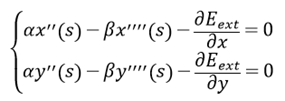将导数用差分近似：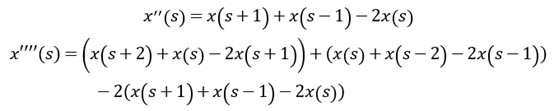记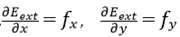则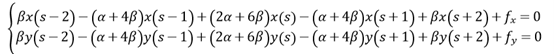写成矩阵形式：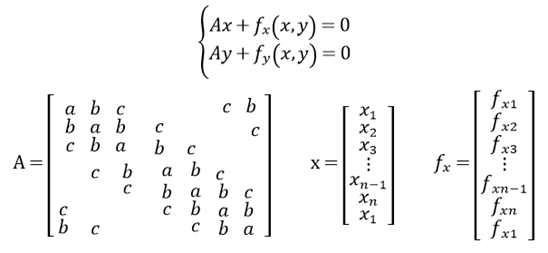A为五对角循环矩阵，a=2α+6β，b=-(α+4β)，c=β 。
利用梯度下降法，可得解得：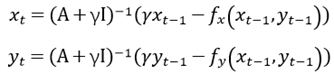3. 代码
使用说明：

matlab编写，需要在matlab下运行。matlab文件路径下需要有一个名为 test.jpg 的 uint8 类型的二维灰度图，该图片即为需要分割的图片。运行时，在matlab命令行直接输入m文件名称，然后将会显示出  test.jpg  图片，需要在该图片上手动画出初始轮廓。画初始轮廓线时，鼠标点击图片中的几个位置，程序会以直线段的方式将这个几个点连接起来，作为初始轮廓线。画好后，关闭图片，然后在命令行按 enter 键，让程序继续运行。 未设置迭代的终点，默认为400次迭代，若没有收敛自行更改迭代次数。
代码如下：
% 基本Snake活动轮廓模型
I=imread(‘test.jpg’);   % 读入的图片应为uint8类型二维的灰度图
snake(I);			    % 对图像I求其中需要分割物体的snake边界
function snake(I)
% Snake主体部分
alpha=0.5; beta=0;      % 连续参数alpha=0.5；平滑参数beta=0；步长为1
[x,y]=DrawLine(I);	% 在图像I上手动画线，得到初始轮廓线
a=2*alpha+6*beta; b=-(alpha+4*beta); c=beta;
J=[c b a b c]; h=max(size(x));
A=diagCyclMat(h,J);     % 求取设定参数下的五对角循环矩阵
II=eye(h); [m,~]=size(I);        % 初始化
I=double(I);
I1=-ff(I);                       % 高斯势能I1
[I2x,I2y]=NGradient(I1);         % I1的负梯度I2
T=max(max(abs(I2x(😃)),max(abs(I2y(😃)));
I2x=I2x/T; I2y=I2y/T;            % 梯度归一化
fx=-1*I2x; fy=-1*I2y;            % f为图像I的高斯势能的梯度
for t=1:400                      % 迭代，未计算迭代终点
ffx=fx(m*(uint16(x)-1)+uint16(y));
ffy=fy(m*(uint16(x)-1)+uint16(y));
x=((II/(A+II))(x’-ffx’))’;
y=((II/(A+II))(y’-ffy’))’;
end
I=uint8(I); imshow(I);  hold on
plot(x,y,‘Color’,‘White’)         % 显示最终Snake轮廓线
end
function I1=ff(I)
%求取I的边缘函数（负高斯势能）
%5阶Standard Deviation=3的高斯滤波，sobel梯度
h=fspecial(‘gaussian’,5,3); w1=fspecial(‘sobel’); w2=w1’;
Is=imfilter(double(I),h,‘conv’,‘replicate’);
I1=imfilter(Is,w1,‘replicate’).^2+imfilter(Is,w2,‘replicate’).^2;
end
function [I2x,I2y]=NGradient(I)
%求取I的负梯度
%sobel梯度
w1=fspecial(‘sobel’); w2=w1’;
I=double(I);
I2y=imfilter(I,w1,‘replicate’);
I2x=imfilter(I,w2,‘replicate’);
end
function A=diagCyclMat(n,J)
% A = diagonal cycle(J) matrix.
%生成一个以向量J为循环体的对角循环矩阵
%2017.10.27
l=length(J); h=(l+1)/2;
if n<l
error(‘A is too small to hold J’);
end
if mod(l,2)==0
error(‘length.J is not odd’);
end
A=zeros(n);
for i=1:n
j=i;
A(i,j)=J(h);
k=1;
while (h-k)~=0
if (j-k)<1
j=j+n;
end
A(i,j-k)=J(h-k);
k=k+1;
end
k=1;
while (h+k)~=l+1
if (j+k)>n
j=j-n;
end
A(i,j+k)=J(h+k);
k=k+1;
end
end
end
function [x,y]=DrawLine(I)
imshow(I)
hold on
tag=0; P=zeros(2); LX=[]; LY=[];
set(gcf,‘WindowButtonDownFcn’,@DoLine);
pause;
x=LX; y=LY;
function DoLine(,)
pt=get(gca,‘CurrentPoint’);
if tag==0
P(1,1)=pt(1,1); P(1,2)=pt(1,2);
tag=1;
else
P(2,1)=pt(1,1); P(2,2)=pt(1,2);
LinkLine§;
P(1,1)=P(2,1); P(1,2)=P(2,2);
end
end
function LinkLine§
xh=abs(P(2,1)-P(1,1));
yh=abs(P(2,2)-P(1,2));
if yh>xh
n=int16(yh+1);
k=double((P(2,1)-P(1,1))/(P(2,2)-P(1,2)));
k1=(P(2,2)-P(1,2))/yh;
X=zeros(1,n);Y=zeros(1,n);
for i=1:n
Y(i)=P(1,2)+(i-1)k1;
X(i)=P(1,1)+ceil((i-1)k1k);
end
else
n=int16(xh+1);
k=double((P(2,2)-P(1,2))/(P(2,1)-P(1,1)));
k1=(P(2,1)-P(1,1))/xh;
X=zeros(1,n);Y=zeros(1,n);
for i=1:n
X(i)=P(1,1)+(i-1)k1;
Y(i)=P(1,2)+ceil(kk1(i-1));
end
end
LX=[LX X]; LY=[LY Y];
plot(LX,LY,‘Color’,‘Red’)
hold on
end
end

转载自：https://blog.csdn.net/caifang112/article/details/79884126


展开全文• 包含源代码及适用图片，可运行.是基于图像的梯度信息进行处理的，属于水平集范畴-Measured the active contour model code is based on the gradient of the image information processing, belonging to the scope ...
• 活动形状模型（Active Shape Models）与活动轮廓模型（Active Contour Models）的MATLAB实现。ASM
• 基本Snake活动轮廓模型，matlab，代码，基本Snake活动轮廓模型
• 经典的GAC 活动轮廓模型，matlab代码，比较全。但是效率有点慢。
• snake活动轮廓边界提取源码 MATLAB程序
• ## 活动轮廓模型——CV模型

万次阅读 热门讨论 2018-05-29 20:02:59
活动轮廓模型——CV模型原理：效果图matlab部分代码：clc clear all; close all; addpath('other methods\'); %% path of saving outDir1 = 'test'; mkdir(outDir1); outDir2 = 'results\ChanVese'; mkdir(outDir2);...
活动轮廓模型——CV模型原理：见论文http://www.sfu.ca/~rpyke/research/vese_chan.pdf博客：https://blog.csdn.net/u011460059/article/details/59538983效果图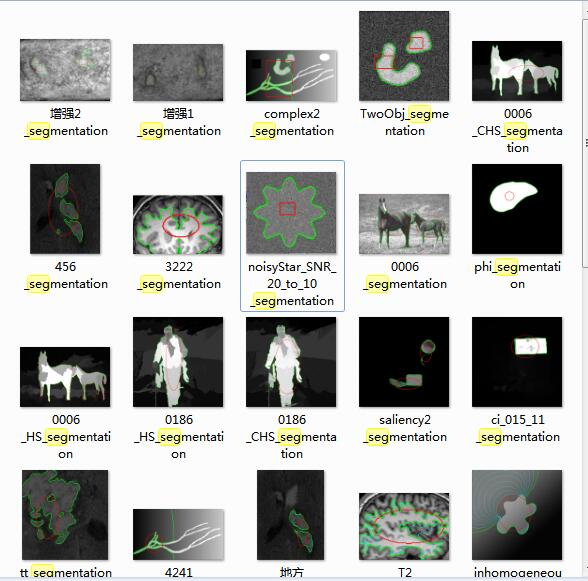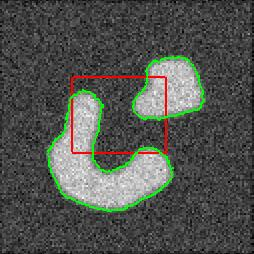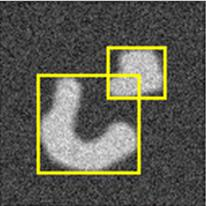matlab部分代码：clc
clear all;
close all;
addpath('other methods\');
%% path of saving
outDir1 = 'test';
mkdir(outDir1);
outDir2 = 'results\ChanVese';
mkdir(outDir2);
outDir3 = 'results\中间过程';
mkdir(outDir3);
%% input an image
[filename, filepath] = uigetfile({'*.jpg;*.ppm; jpeg *.;*.bmp;*.png;*.gif'},'Choose Input Image');
if isequal(filename,0) || isequal(filepath,0)
disp('User pressed cancel')
return
else
fullfp = fullfile(filepath, filename);
end
Img = imread(fullfp);
imwrite(Img,strcat(outDir1,'\',filename));%save the original image

%% RGB image-->Gray image
if length(size(Img)) > 2
Img = rgb2gray(Img);
end
figure(1),imshow(Img,'InitialMagnification',200);

%% set parameters and draw initial contour
img_magnify = 200;
mask_type   = 'rect';  %% mask_type = 'rect' or  'ellipse' or 'multiball'
num_iter    = 400;  %% may be revised!
color       = 'g';     %% the color of the final contour
thresh      = 0;
length_reg  = 0.4;
display_interval = 50;
acObj = GeomAC(im2double(Img),img_magnify,num_iter,color,thresh,mask_type);
acObj.initRegions();  %% Initialize the level set
acObj.computeSDF();   %% Compute the signed distance function

%% Initial level set function
figure(2);
mesh(acObj.phi0);   % for a better view, the LSF is displayed upside down
hold on;
title('Initial level set function');
view([-75 60]);
box on
saveas(gcf,strcat(outDir2,'\',strtok(filename,'.'),'_initial contour.bmp'));
hold off

%% compare experiments
% load('ImgMask3.mat');
% phi0 = 1-u;

u =1 - acObj.init_reg;
str = strcat(strtok(filename,'.'),'_mask');
save ImgMask u;

%% compare with other level set methods
tic;
intermed_phi = acObj.runChanVese(length_reg,display_interval);     % regularization-factor,display-interval
toc;

%% Final level set function
figure(4);
[aa,bb,cc] = size(intermed_phi);
mesh(intermed_phi(:,:,cc)'); % for a better view, the LSF is displayed upside down
hold on;  contour(intermed_phi(:,:,cc)', [0,0], 'g','LineWidth',2);
title('Final level set function');
view([60 45]);
box on
saveas(gcf,strcat(outDir2,'\',strtok(filename,'.'),'_final contour.bmp'));

%% Show Intermediate results
[~,~,l] = size(intermed_phi);
last = l-2;
figure(5);imshow(im2double(Img),'InitialMagnification',200); hold on;
%figure(5);imshow(uint8(Img),'InitialMagnification',200); hold on;
for ii = 1 : last
if ii == 1       %-- Initial Contour
GeomAC.showIntermediate(acObj.phi0(:,:,ii),'r',2);
%GeomAC.showIntermediate(phi0(:,:,ii),'r',2);
elseif ii == last   %-- Final Contour
GeomAC.showIntermediate(intermed_phi(:,:,ii),'g',2);
%     else             %-- Intermediate output
%        GeomAC.showIntermediate(intermed_phi(:,:,ii),'c',0.2);
end
end
hold off;

%%%%%%%%%%% save fig
[m,n] = size(Img);
set(gca,'units','pixels','Visible','off');
q=get(gca,'position');
q(1)=0;%设置左边距离值为零
q(2)=0;%设置右边距离值为零
set(gca,'position',q);

%frame=getframe(gcf,[0,0,n,m]);%% for medical images
frame=getframe(gcf,[0,0,2*n,2*m]);

im=frame2im(frame);
imwrite(im,strcat(outDir2,'\',strtok(filename,'.'),'_segmentation.bmp'));

%% Test Heaviside-Dirac-gradient relationship
Dirac_global    = @(x,e) ((e/pi)./(e^2.+ x.^2));
Heaviside       = @(y,e) (0.5*(1+(2/pi)*atan(y/e)));

phi = acObj.phi;
eps = 2;

h = Heaviside(phi,eps);
[hx,hy]=gradient(h);
g_h = sqrt(hx.^2+hy.^2);
delta = Dirac_global(phi,eps);

figure(6);
subplot(2,2,1); imshow(phi); title('\phi');
subplot(2,2,2); imshow(h); title('H(\phi)');
subplot(2,2,3); imshow(g_h); title('|\nabla H(\phi)|');
subplot(2,2,4); imshow(delta); title('\delta(\phi)');
saveas(gcf,strcat(outDir2,'\',strtok(filename,'.'),'_compare.bmp'));
%% Show Intermediate results
inter_res = intermed_phi;
[~,~,l] = size(inter_res);
last = l-2;
for ii = 1 : last
figure(4),imshow(im2double(Img),'InitialMagnification',200); hold on;
if ii == 1       %-- Initial Contour
showIntermediate(acObj.phi0(:,:,ii),'r',2);

%%%%%%%%%%%%%%%%%%%%%%%%%%%%%%%%%%%%%%%%%%%
set(gca,'units','pixels','Visible','off');
q=get(gca,'position');
q(1)=0;
q(2)=0;
set(gca,'position',q);

frame=getframe(gcf,[0,0,2*n,2*m]);
im=frame2im(frame);
imwrite(im,strcat(outDir3,'\',number2string(ii),'.bmp'));

else
showIntermediate(inter_res(:,:,ii),'g',2);

%%%%%%%%%%%%%%%%%%%%%%%%%%%%%%%%%%%%%%%%%%%%
set(gca,'units','pixels','Visible','off');
q=get(gca,'position');
q(1)=0;
q(2)=0;
set(gca,'position',q);

frame=getframe(gcf,[0,0,2*n,2*m]);
im=frame2im(frame);
imwrite(im,strcat(outDir3,'\',number2string(ii),'.bmp'));
hold off
end
end
%%  画矩形框
figure(7);
evolved_u = phi;
finalResult = double(im2bw(evolved_u));
imshow(uint8(Img));
hold on;
[m,n]=size(finalResult);

L=bwlabel(finalResult);
stats=regionprops(L,'all');

set(gcf,'color','w');
set(gca,'units','pixels','Visible','off');
q=get(gca,'position');
q(1)=0;q(2)=0;
set(gca,'position',q);

for i=1:length(stats)
title('分割效果');
hold on;
rectangle('position',stats(i).BoundingBox,'edgecolor','y','linewidth',2);
temp = stats(i).Centroid;
drawnow;
end

frame=getframe(gcf,[0,0,n,m]);
im=frame2im(frame);
%imwrite(im,strcat(outDir,'\',strtok(filename,'.'),'_seg.bmp')); % 保存分割效果图
imwrite(im,'segResult.bmp'); % 保存分割效果图
%% delete acObj
% delete(acObj);
% close all;
展开全文CV模型 水平集方法
• 此示例显示了如何使用活动轮廓(snakes)执行三维分割。您可以使用the Volume Viewer app查看结果 一、准备数据 将人体胸部CT扫描数据加载到工作空间中。要运行此示例，您必须使用附加浏览器从MathWorks下载样本数据...
目录：

一、准备数据

二、肺部分割

三、创建种子MASK掩膜并使用活动轮廓（snakes）分割肺部

四、计算分割肺的体积

例程完整源码：

参考链接

本例程配套完整源码和数据下载

此示例显示了如何使用活动轮廓(snakes)执行三维分割。您可以使用the Volume Viewer app查看结果

一、准备数据

将人体胸部CT扫描数据加载到工作空间中。要运行此示例，您必须使用附加浏览器从MathWorks下载样本数据。

使用加载项资源管理器安装示例数据

图像处理工具箱使样本3-D MRI (三维磁共振)胸部扫描数据集作为一个可选功能。要获取此数据集，请使用附加组件浏览下载它

1、打开Matalb——>主页——>选择附加功能 ——> 获取附加功能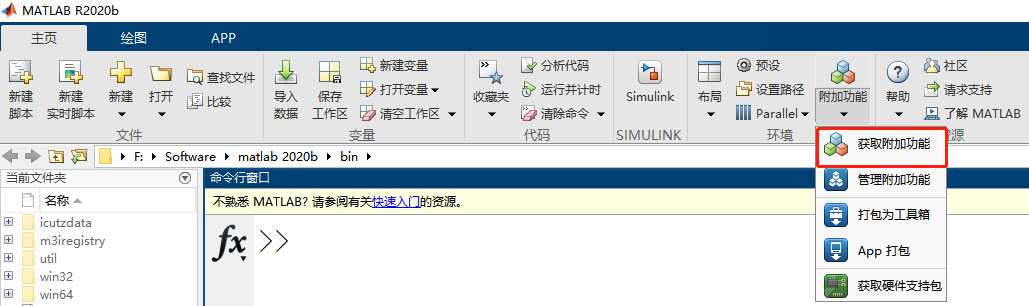2、在打开的附加功能资源管理器 中搜索 Image Processing Toolbox Image Data3、在搜索结果中单击数据包——>安装。遵循安装人员提供的说明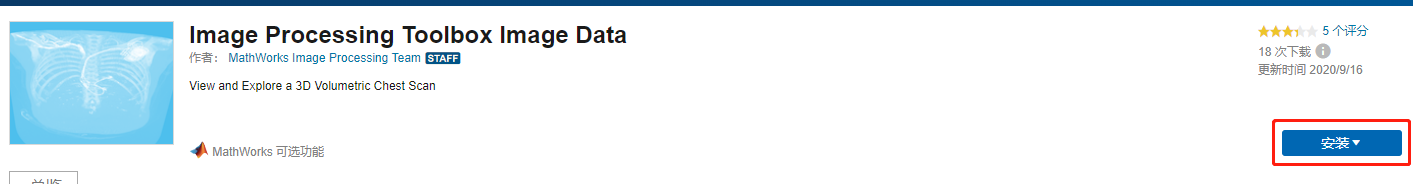4、下载完成后得到的安装文件夹MathWorks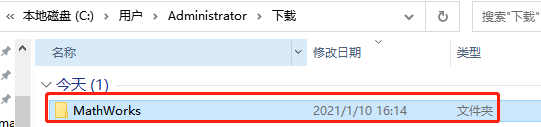5、在Windows平台进行安装：

在Matlab的安装目录下选中目录——>键盘cmd——>回车输入SupportSoftwareInstaller.exe -archives C:\Users\Administrator\Downloads\MathWorks\SupportPackages\R2020b\

（注Matlab2017----Matlab2020版本可能是install_supportsoftware.exe）还有后面刚下载安装文件夹目录自行更改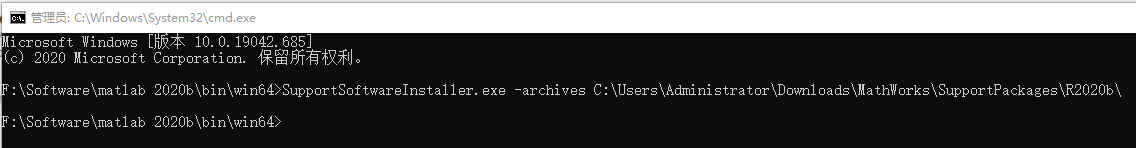勾选——>下一步——>我接受——>下一步——>安装完毕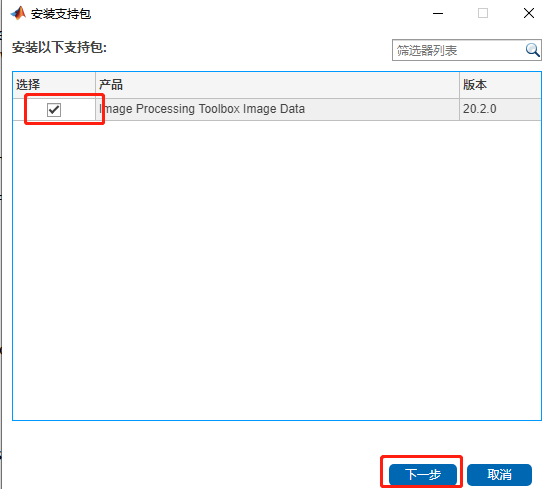6、关闭Matlab后重新打开，命令行窗口输入

load chestVolume
whos

加载数据到Matlab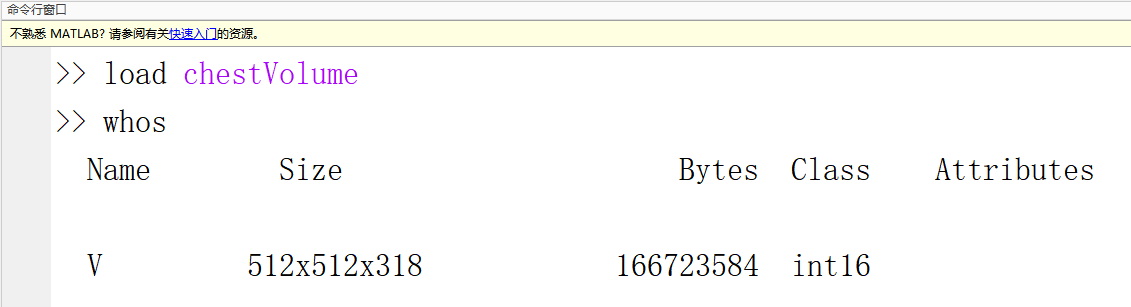7、将CT扫描数据从int16转换为single，以将值标准化到范围[0，1]

V = im2single(V);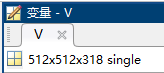8、使用matlab中 APP——> 图像处理与计算机视觉——>Volume Viewer查看胸部扫描。也可以在命令行窗口使用volumeViewer(V)命令打开应用程序。使用ct-骨骼(ct-bone)，获得胸部扫描的最佳视图

volumeViewer(V)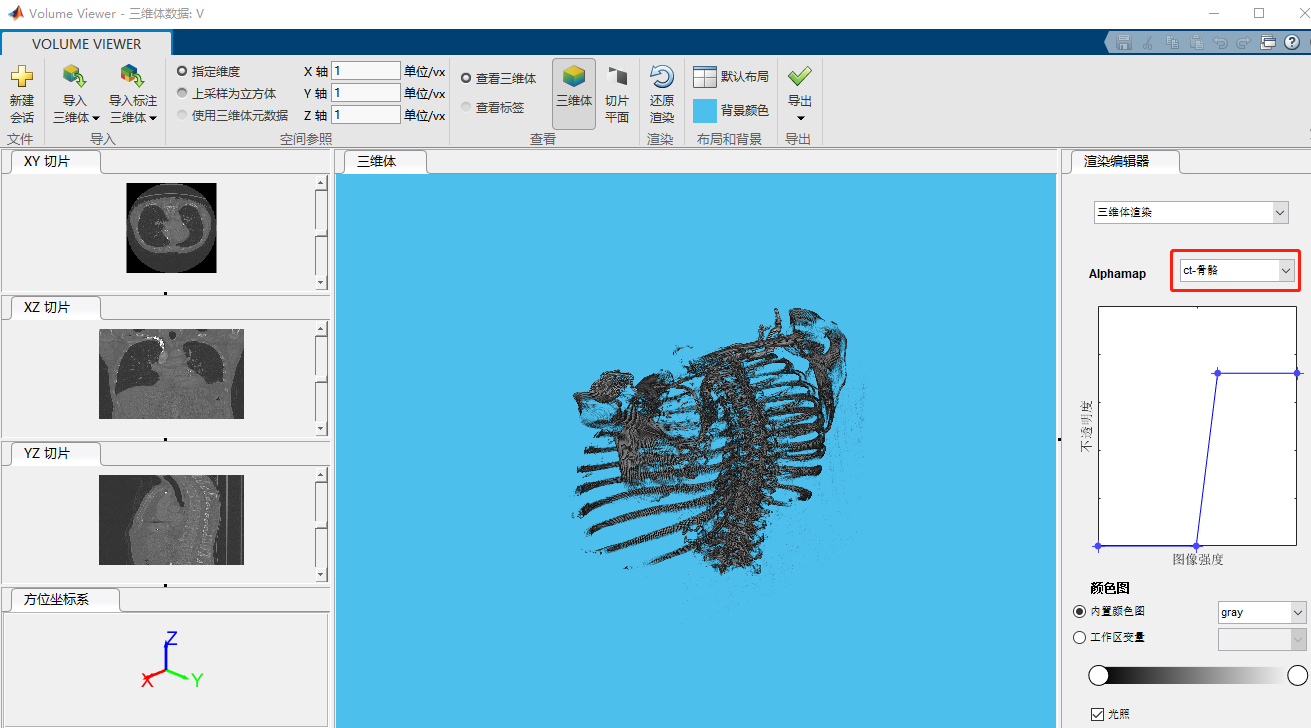二、肺部分割

使用活动轮廓(active contour)技术对CT扫描数据进行肺部分割。主动轮廓是一种区域生长算法，需要初始种子点(initial seed points)。该示例使用图像分割APP通过分割两个正交的二维切片来创建种子掩膜(seed mask)，一个在XY平面，另一个在XZ平面。然后，该示例将这两个分段插入到三维掩膜(3-D mask)中。然后将该遮罩传递给活动轮廓函数，以创建胸腔中肺部的三维分割。(此示例使用活动轮廓方法，但您也可以使用其他分割技术来实现相同的目标，例如flood-fill。

1、在XY和XZ维度提取中心切片

XY = V(:,:,160);
XZ = squeeze(V(256,:,:));   %squeeze函数三维转化为二维切片，即去除一个维度

2、使用图像显示功能查看二维切片

查看XY切片

figure
imshow(XY,[],'Border','tight');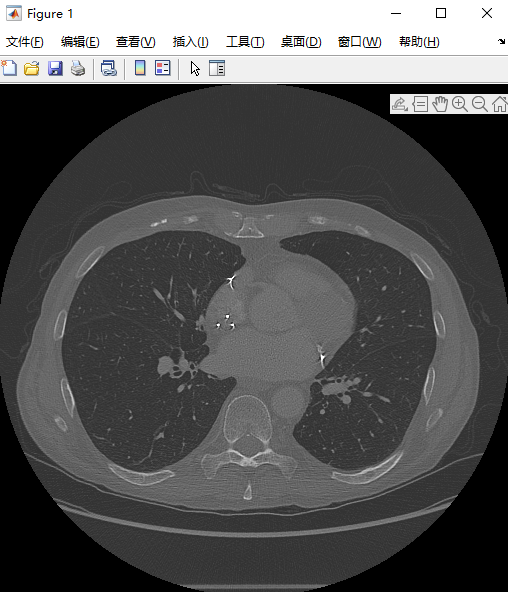查看XZ切片

imshow(XZ,[],'Border','tight');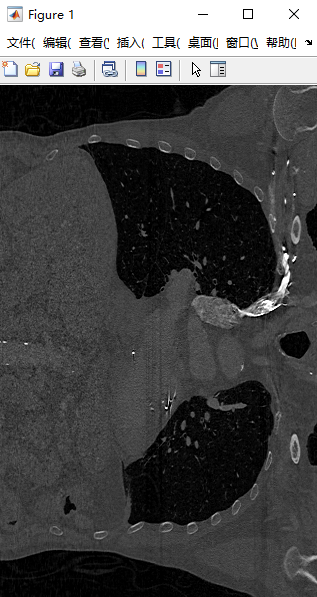3、可以在matlab中 APP——> 图像处理与计算机视觉——>Image Segmenter 中执行分割。或使用图像分割器命令，指定一个二维切片作为参数进行分割。如：imageSegmenter(XY)

imageSegmenter(XY)   %对XY切片进行分割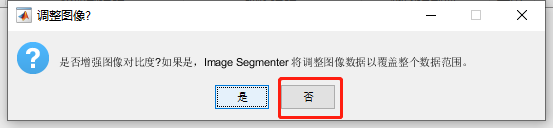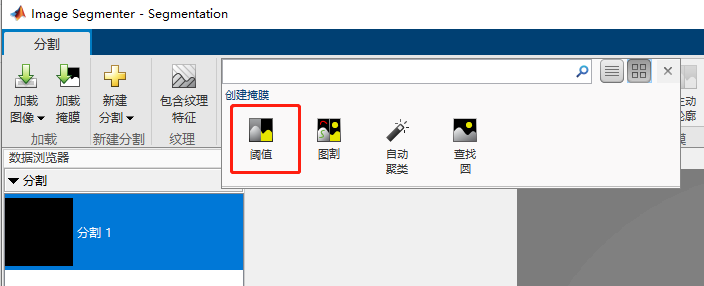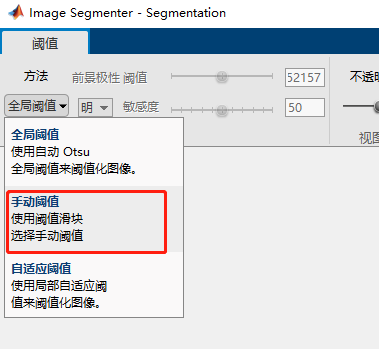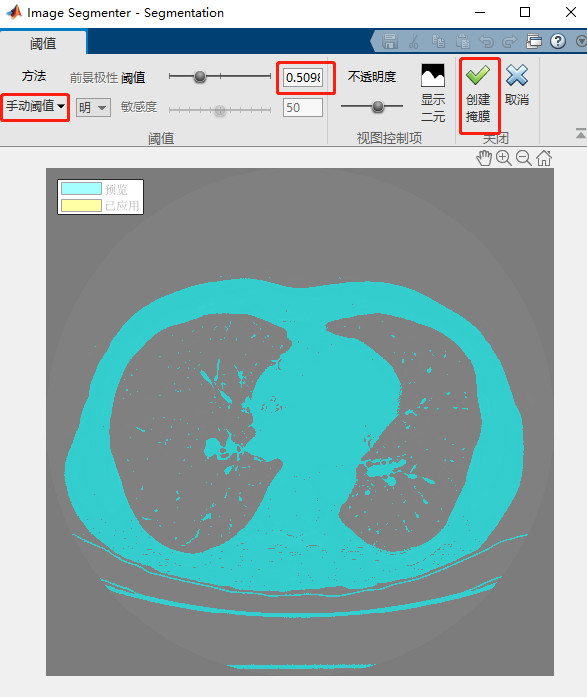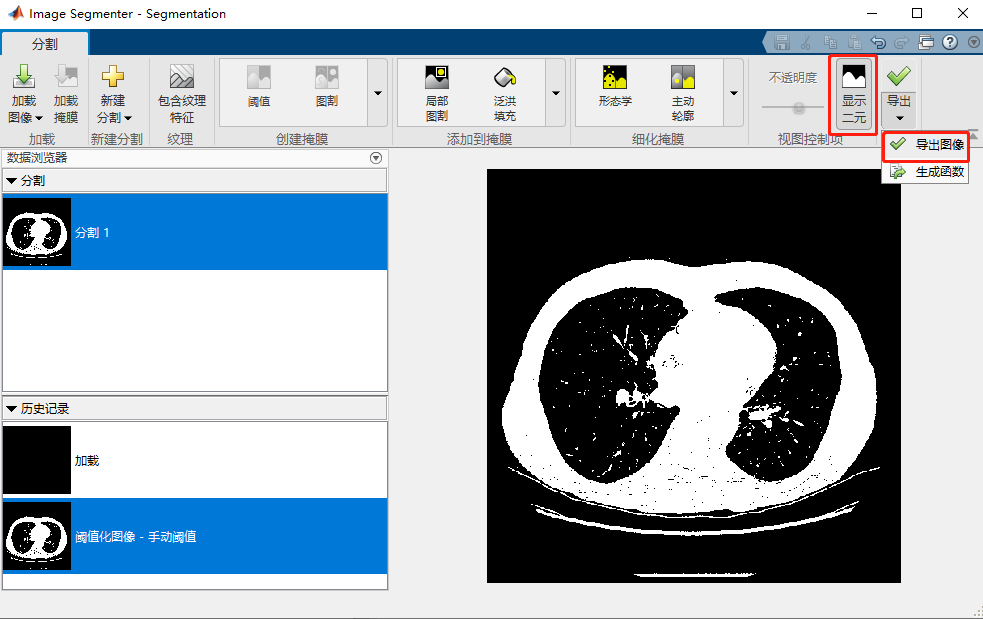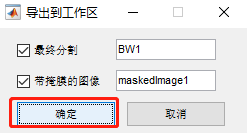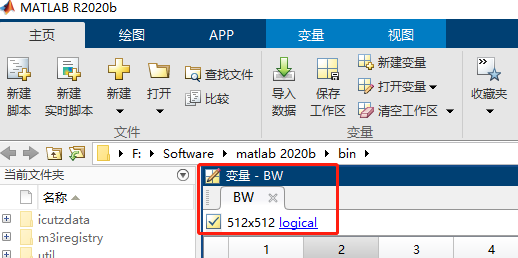4、第3小节实现也可以通过命令行实现

BW = XY > 5.098000e-01;   %提取XY切片中阈值大于0.5098的像素，并保存为二值图像BW5、在这个初始肺部分割之后，使用 APP——> 图像处理与计算机视觉——>Image Segmenter——>细化掩膜菜单中的选项来对掩膜BW进行进一步优化

加载掩膜：将粗分割的肺部掩膜加载到Image Segmenter APP中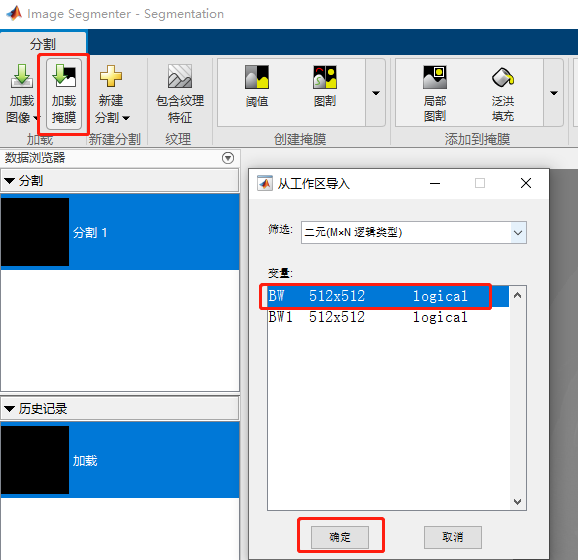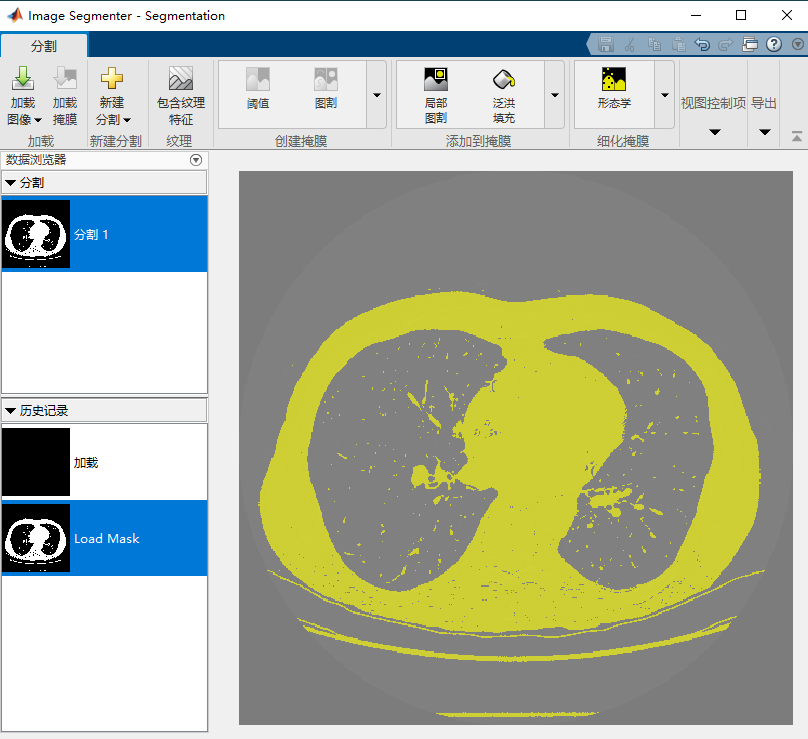反转掩膜(Invert Mask)：反转掩膜图像，以便肺部位于前景中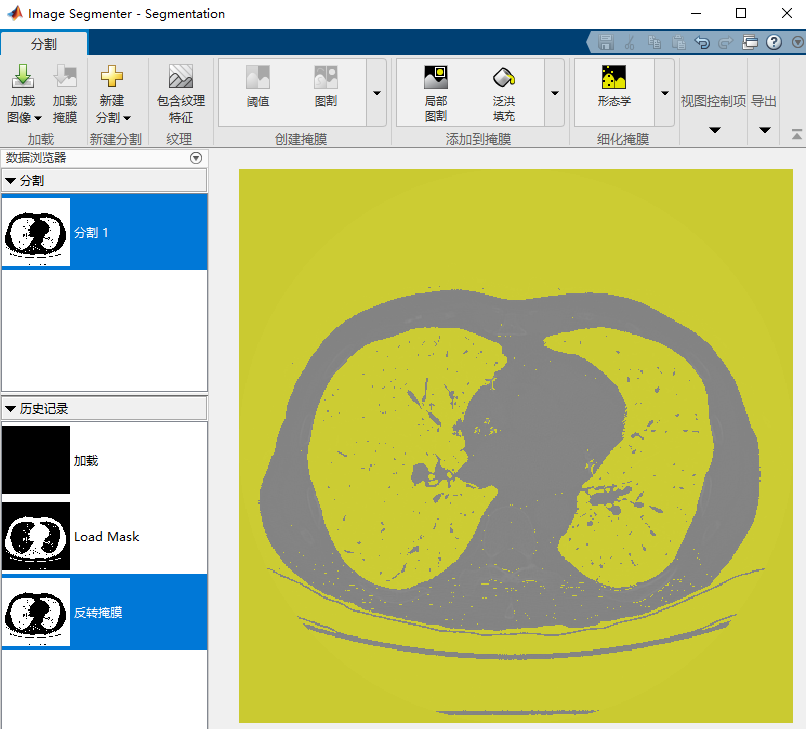清理边框(Clear Borders)：移除除肺部之外的其他分割杂质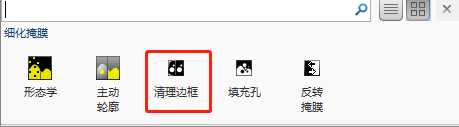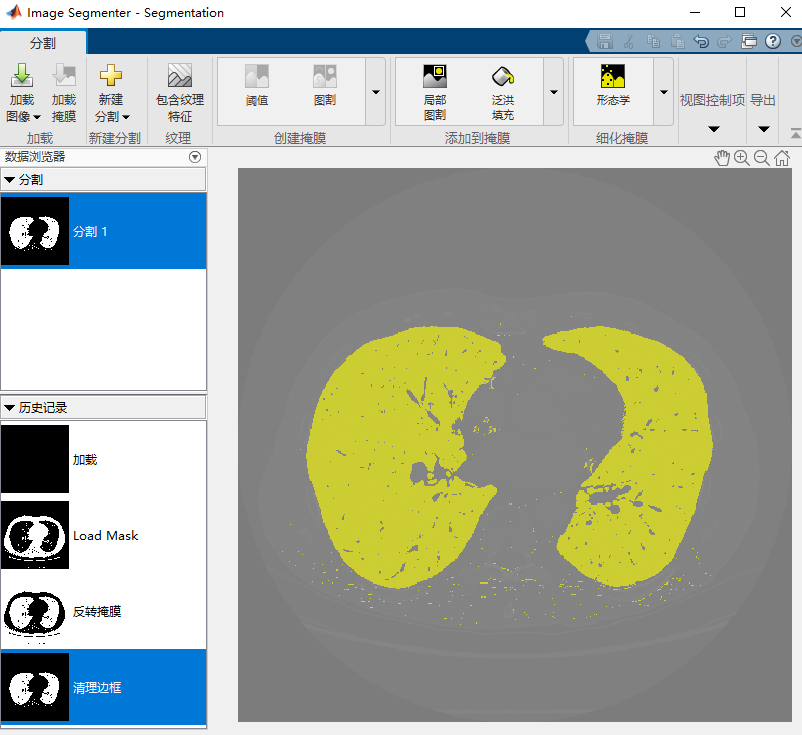填充孔(Fill Holes)：填充肺部分割内的孔洞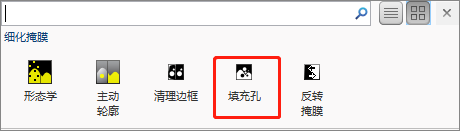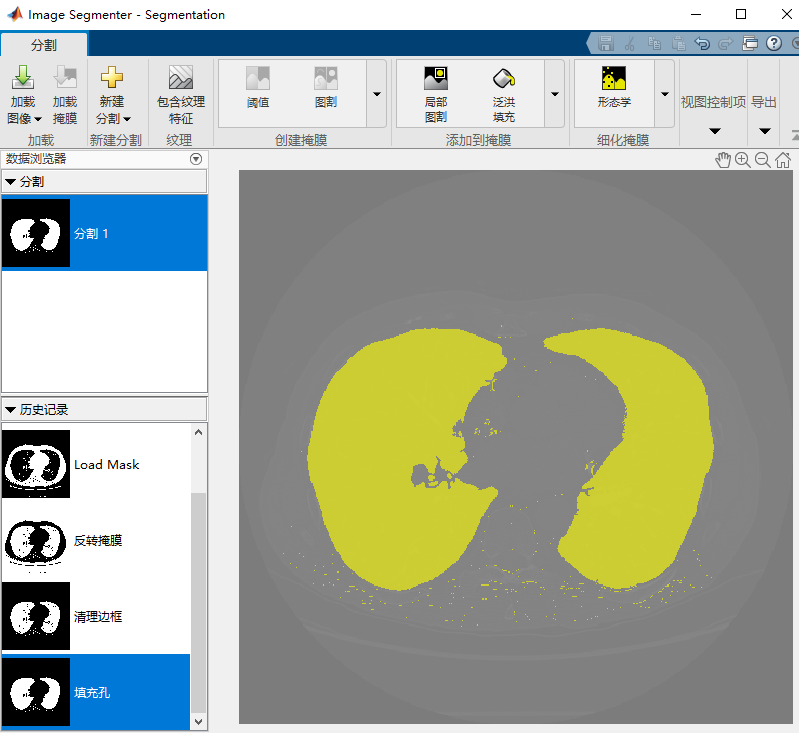形态学(Morphology)：使用形态学选项来平滑肺部分割的边缘

选择“形态学”——>选择腐蚀“erode”——>应用——>关闭形态学——>显示二元——>导出

便可将优化后的掩膜图像保存到工作区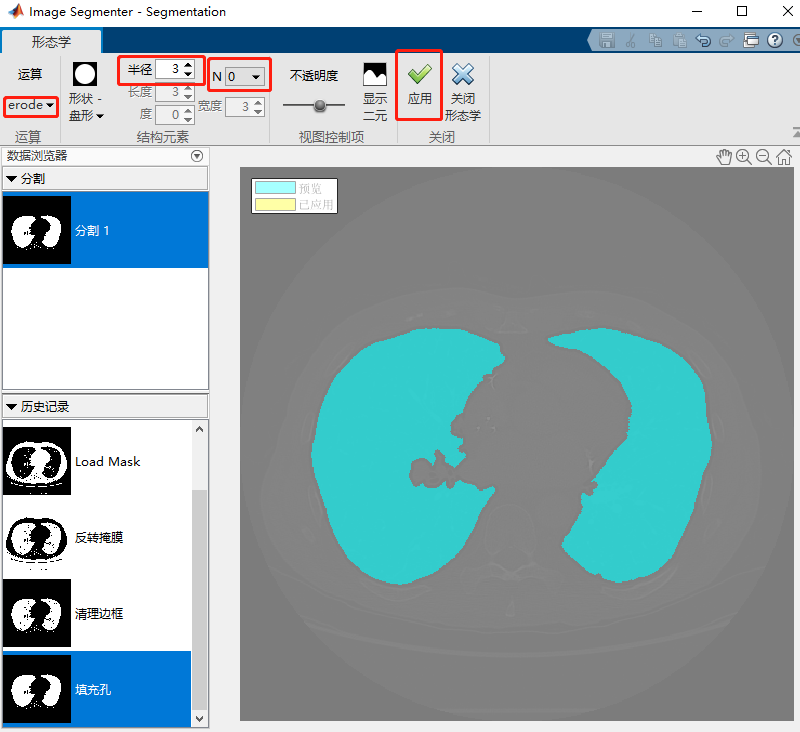形态学处理后二元图（即二值化图）：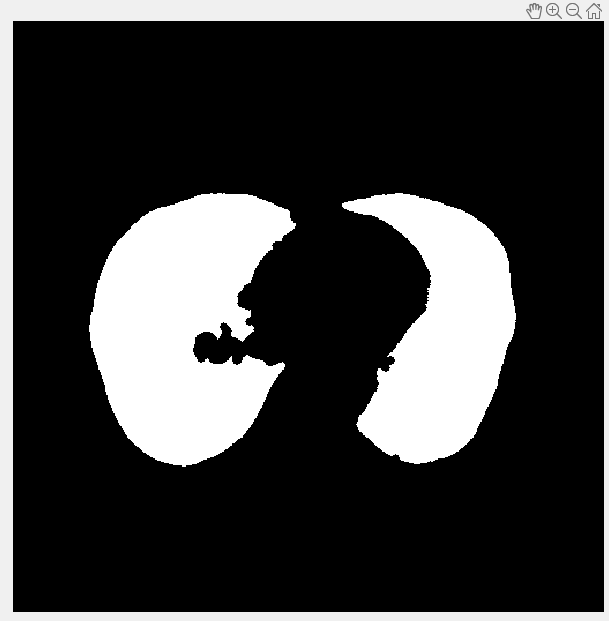第5小节也可以通过源码来实现：

BW = imcomplement(BW);    %反转掩膜（使肺部位于前景中）
BW = imclearborder(BW);   %清理边框（移除除肺部之外的其他分割杂质）
BW = imfill(BW, 'holes'); %填充孔（填充肺部分割内的孔洞）
%形态学处理
radius = 3;     %设置结构元素半径为3
decomposition = 0;
se = strel('disk',radius,decomposition);  %创建盘形结构元素
BW = imerode(BW, se);   %形态学的腐蚀操作，得到的结果图像覆盖为BW
maskedImageXY = XY;     %XY切片赋值给maskedImageXY（即复制一个图像maskedImageXY）
maskedImageXY(~BW) = 0; %将maskedImageXY中BW图像中的非1区域都清0（即只保留maskedImageXY图像中的肺部区域）
imshow(maskedImageXY)   %显示图像maskedImageXY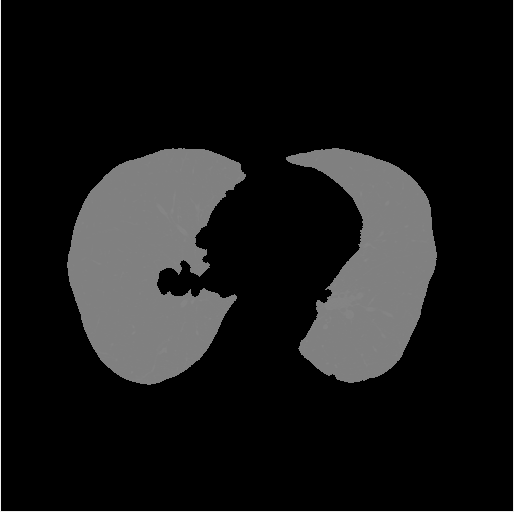6、XZ切片的掩膜优化处理

在XZ切片上执行上述相同的操作。对于XZ切片，全局阈值可创建了一个适当的分段(调用imbinarize)。与XY切片一样，在侵蚀操作中，指定半径为13以移除无关的小对象

BW = imbinarize(XZ);      %对XZ切片进行全局阈值提取
BW = imcomplement(BW);    %反转掩膜（使肺部位于前景中）
BW = imclearborder(BW);   %清理边框（移除除肺部之外的其他分割杂质）
BW = imfill(BW,'holes');  %填充孔（填充肺部分割内的孔洞）
%形态学处理
radius = 13;              %设置结构元素半径为13
decomposition = 0;
se = strel('disk',radius,decomposition);  %创建盘形结构元素
BW = imerode(BW, se);     %形态学的腐蚀操作，得到的结果图像覆盖为BW
maskedImageXZ = XZ;       %XZ切片赋值给maskedImageXZ（即复制一个图像maskedImageXZ）
maskedImageXZ(~BW) = 0;   %将maskedImageXZ中BW图像中的非1区域都清0（即只保留maskedImageXZ图像中的肺部区域）
imshow(maskedImageXZ)     %显示图像maskedImageXZ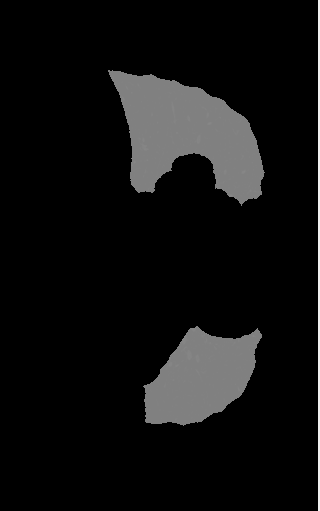三、创建种子MASK掩膜并使用活动轮廓（snakes）分割肺部

创建三维种子掩膜mask，并使用活动轮廓功能来分割肺部。创建与输入体积大小相同的逻辑三维体积（volume），并在适当的空间位置插入掩膜_XY和掩膜_XZ

%创建3-D种子mask
mask = false(size(V));    %创建与V矩阵维数大小相同的，元素都为0矩阵mask作为3-D种子mask
mask(:,:,160) = maskedImageXY; %maskedImageXY中的值赋值给mask的第三维度Z的第160切片
%reshape将maskedImageXZ重构为1*512*318矩阵，再与mask的第一维度切片进行或运算，目的同XY切片
mask(256,:,:) = mask(256,:,:)|reshape(maskedImageXZ,[1,512,318]);

使用此三维种子掩膜mask，通过活动轮廓方法（snakes）在三维体积中分割肺部。此操作可能需要几分钟时间。要获得高质量的分割，可使用直方图均衡化（histeq）在可用范围内扩展体素值

%使用活动轮廓方法（snakes）在三维体积中分割肺部，得到三维二值分割结果BW，三维原数据分割结果segmentedImage
V = histeq(V);             %对原始数据V进行直方图均衡化，增强对比度
BW = activecontour(V,mask,100,'Chan-Vese'); %调用活动轮廓方法，设置迭代100次，得到分割二值三维图像BW
segmentedImage = V.*single(BW);             %原数据V与分割二值数据BW进行点乘运算，将分割数据部分以原数据像素显示

之后可以通过运行命令volumeViewer(segmentedImage)可在Volume Viewer APP中查看三维分段肺效果。通过操纵渲染编辑器中的alphamap设置，可以获得肺部的良好视图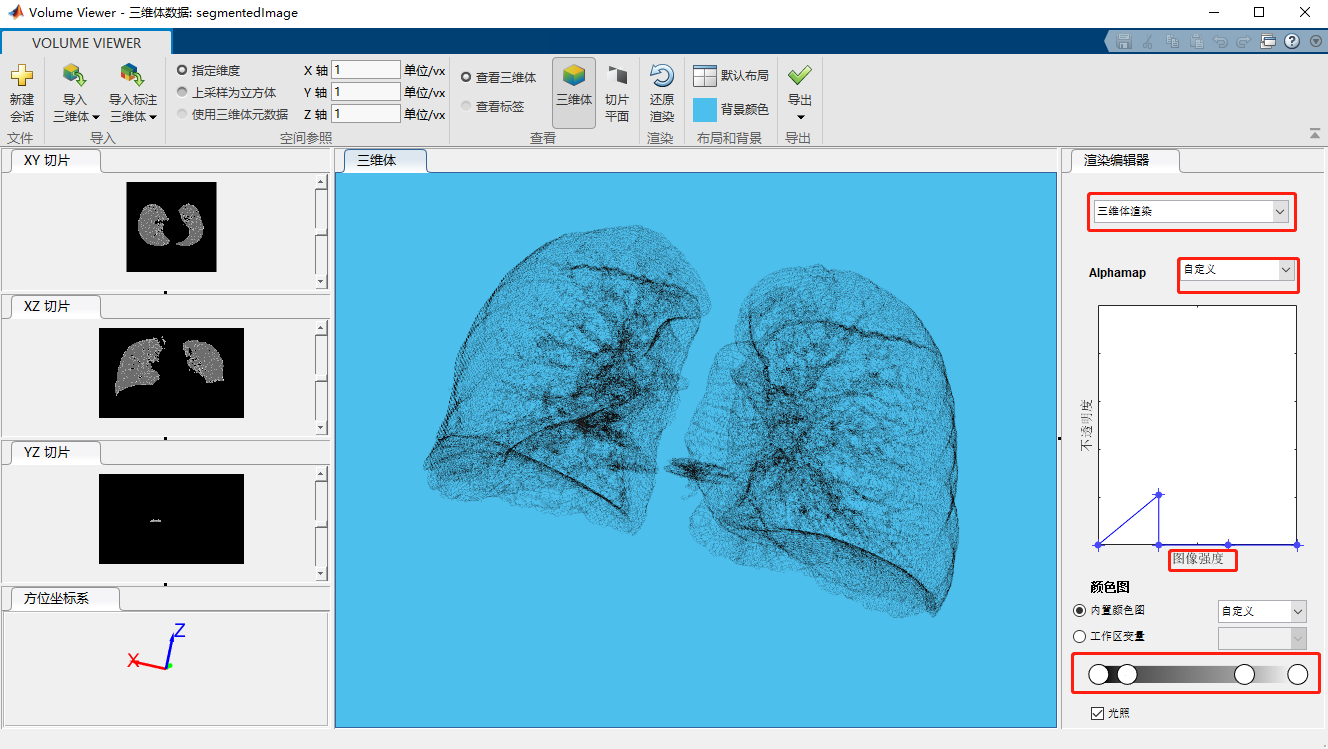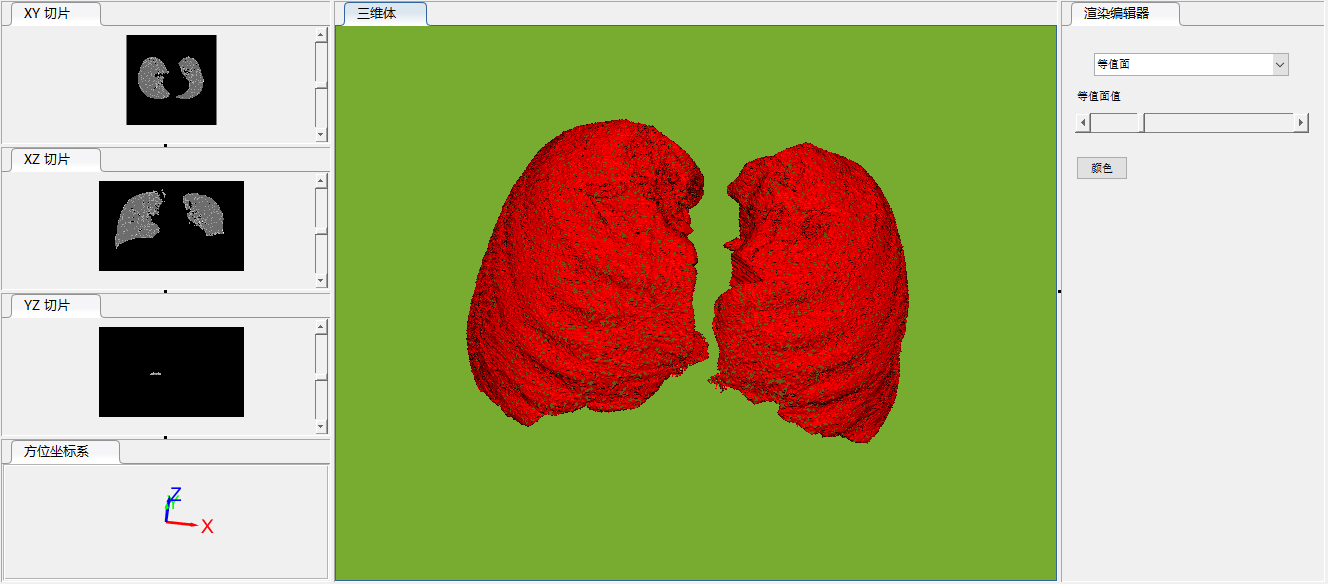四、计算分割肺的体积

1、使用regionprops3函数来计算肺的体积个数

volLungsPixels = regionprops3(logical(BW),'volume'); %计算分割肺的体积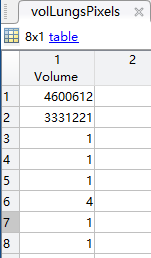2、根据从原始文件元数据中收集的x、y和z维度中体素的间距，计算分割肺的体积大小（单位：）

spacingx = 0.76;     %单个体素（volume）X轴大小（单位mm）
spacingy = 0.76;     %单个体素（volume）Y轴大小（单位mm）
spacingz = 1.26*1e-6;%单个体素（volume）Z轴大小（单位mm）
unitvol = spacingx*spacingy*spacingz;   %单个体素（volume）的体积大小（单位:mm³）

volLungs1 = volLungsPixels.Volume(1)*unitvol;  %左肺总体积大小（单位:mm³）
volLungs2 = volLungsPixels.Volume(2)*unitvol;  %右肺总体积大小（单位:mm³）
volLungsLiters = volLungs1 + volLungs2         %分割肺部总体积大小（单位:mm³）

单个体素（volume）体积大小unitvol为7.2778*10^-7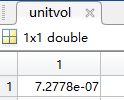分割肺部所有体素（volume）总体积大小volLungsLiters为5.7726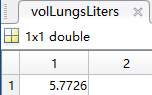注：由于官方例程并未给定单个体素的大小单位，所有我觉得单个体素Z轴大小应该是有问题的

例程完整源码：

close all,clear all; %清空数据
%%%准备数据%%%%%%%%%%%%%%%%%%%
load chestVolume  %加载样本数据
V = im2single(V); %将CT扫描数据V从int16转换为single，以将其值标准化到范围[0，1]
volumeViewer(V);  %使用Volume Viewer APP查看胸部扫描图像

%%%肺部分割%%%%%%%%%%%%%%%%%%%
%在XY和XZ维度提取中心切片
XY = V(:,:,160);
XZ = squeeze(V(256,:,:));   %squeeze函数三维转化为二维切片，即去除一个维度
figure(1),
imshow(XY,[],'Border','tight'); %使用图像显示功能查看二维切片XY
figure(2),
imshow(XZ,[],'Border','tight'); %使用图像显示功能查看二维切片XZ

%%%创建掩膜Mask_XY
%imageSegmenter(XY);   %对XY切片进行分割
%阈值提取
BW = XY > 5.098000e-01;   %提取XY切片中阈值大于0.5098的像素，并保存为二值图像BW
%创建XY切片的掩膜图像maskedImageXY
BW = imcomplement(BW);    %反转掩膜（使肺部位于前景中）
BW = imclearborder(BW);   %清理边框（移除除肺部之外的其他分割杂质）
BW = imfill(BW, 'holes'); %填充孔（填充肺部分割内的孔洞）
%形态学处理
radius = 3;     %设置结构元素半径为3
decomposition = 0;
se = strel('disk',radius,decomposition);  %创建盘形结构元素
BW = imerode(BW, se);   %形态学的腐蚀操作，得到的结果图像覆盖为BW
maskedImageXY = XY;     %XY切片赋值给maskedImageXY（即复制一个图像maskedImageXY）
maskedImageXY(~BW) = 0; %将maskedImageXY中BW图像中的非1区域都清0（即只保留maskedImageXY图像中的肺部区域）
imshow(maskedImageXY)   %显示图像maskedImageXY

%创建XZ切片的掩膜图像maskedImageXZ
BW = imbinarize(XZ);      %对XZ切片进行全局阈值提取
BW = imcomplement(BW);    %反转掩膜（使肺部位于前景中）
BW = imclearborder(BW);   %清理边框（移除除肺部之外的其他分割杂质）
BW = imfill(BW,'holes');  %填充孔（填充肺部分割内的孔洞）
%形态学处理
radius = 13;              %设置结构元素半径为13
decomposition = 0;
se = strel('disk',radius,decomposition);  %创建盘形结构元素
BW = imerode(BW, se);     %形态学的腐蚀操作，得到的结果图像覆盖为BW
maskedImageXZ = XZ;       %XZ切片赋值给maskedImageXZ（即复制一个图像maskedImageXZ）
maskedImageXZ(~BW) = 0;   %将maskedImageXZ中BW图像中的非1区域都清0（即只保留maskedImageXZ图像中的肺部区域）
imshow(maskedImageXZ)     %显示图像maskedImageXZ

%创建3-D种子mask
mask = false(size(V));    %创建与V矩阵维数大小相同的，元素都为0矩阵mask作为3-D种子mask
mask(:,:,160) = maskedImageXY; %maskedImageXY中的值赋值给mask的第三维度Z的第160切片
%reshape将maskedImageXZ重构为1*512*318矩阵，再与mask的第一维度切片进行或运算，目的同XY切片
mask(256,:,:) = mask(256,:,:)|reshape(maskedImageXZ,[1,512,318]);

%使用活动轮廓方法（snakes）在三维体积中分割肺部，得到三维二值分割结果BW，三维原数据分割结果segmentedImage
V = histeq(V);             %对原始数据V进行直方图均衡化，增强对比度
BW = activecontour(V,mask,100,'Chan-Vese'); %调用活动轮廓方法，设置迭代100次，得到分割二值三维图像BW
segmentedImage = V.*single(BW);             %原数据V与分割二值数据BW进行点乘运算，将分割数据部分以原数据像素显示

volumeViewer(segmentedImage)        %在Volume Viewer APP中查看分割效果

volLungsPixels = regionprops3(logical(BW),'volume'); %计算整个分割肺的体积volume个数，主要由两部分肺（左右肺）组成

spacingx = 0.76;     %单个体素（volume）X轴大小（单位mm）
spacingy = 0.76;     %单个体素（volume）Y轴大小（单位mm）
spacingz = 1.26*1e-6;%单个体素（volume）Z轴大小（单位mm）
unitvol = spacingx*spacingy*spacingz;   %单个体素（volume）的体积大小（单位:mm³）

volLungs1 = volLungsPixels.Volume(1)*unitvol;  %左肺总体积大小（单位:mm³）
volLungs2 = volLungsPixels.Volume(2)*unitvol;  %右肺总体积大小（单位:mm³）
volLungsLiters = volLungs1 + volLungs2         %分割肺部总体积大小（单位:mm³）


参考链接展开全文• 用于图像分割的自适应扩散流活动轮廓Matlab程序已经调好，自己加载图片即可
• matlab开发-活动生物学家的静磁场。活动轮廓的静磁场
• 但是苦苦没有代码，自己写又写不出来，特地来借助这万能的贴吧，询问各位好心人，有没有关于这种文章大matlab代码，如果有，恳求发一份2443671961@qq.com. 只要是用split bregman 算法处理活动轮廓模型的代码均可。...cv模型 优化 算法
• matlab开发-本地全局活动目标模型。基于SOM的强度不均匀性鲁棒主动轮廓模型
• matlab开发-活动生物学家的卷积气电场力。从VEF模型扩展而来的活动轮廓的有效外力
• Matlab图像处理》part1 Snakes：Active ...图像分割之（五）活动轮廓模型之Snake模型简介 简介 在“图像分割之（一）概述”中咱们简单了解了目前主流的图像分割方法。下面咱们主要学习下基于能量泛函的分割方法。
《Matlab图像处理》part1 Snakes：Active Contour Models 主动轮廓模型
参考博客：
数字图像处理-图像分割：Snake主动轮廓模型
Matlab代码及运行结果

图像分割之（五）活动轮廓模型之Snake模型简介

简介
在“图像分割之（一）概述”中咱们简单了解了目前主流的图像分割方法。下面咱们主要学习下基于能量泛函的分割方法。这里学习下Snake模型简单的知识，Level Set（水平集）模型会在后面的博文中说到。
基于能量泛函的分割方法：
该类方法主要指的是活动轮廓模型（active contour model）以及在其基础上发展出来的算法，其基本思想是使用连续曲线来表达目标边缘，并定义一个能量泛函使得其自变量包括边缘曲线，因此分割过程就转变为求解能量泛函的最小值的过程，一般可通过求解函数对应的欧拉(Euler．Lagrange)方程来实现，能量达到最小时的曲线位置就是目标的轮廓所在。
主动轮廓线模型是一个自顶向下定位图像特征的机制，用户或其他自动处理过程通过事先在感兴趣目标附近放置一个初始轮廓线，在内部能量（内力）和外部能量（外力）的作用下变形外部能量吸引活动轮廓朝物体边缘运动，而内部能量保持活动轮廓的光滑性和拓扑性，当能量达到最小时，活动轮廓收敛到所要检测的物体边缘。
一、曲线演化理论

曲线演化理论在水平集中运用到，但我感觉在主动轮廓线模型的分割方法中，这个知识是公用的，所以这里我们简单了解下。
曲线可以简单的分为几种：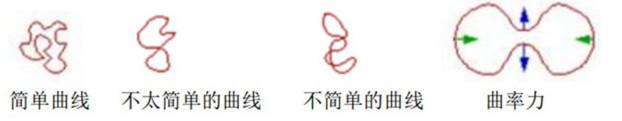曲线存在曲率，曲率有正有负，于是在法向曲率力的推动下，曲线的运动方向之间有所不同：有些部分朝外扩展，而有些部分则朝内运动。这种情形如下图所示。图中蓝色箭头处的曲率为负，而绿色箭头处的曲率为正。
简单曲线在曲率力（也就是曲线的二次导数）的驱动下演化所具有的一种非常特殊的数学性质是：一切简单曲线，无论被扭曲得多么严重，只要还是一种简单曲线，那么在曲率力的推动下最终将退化成一个圆，然后消逝（可以想象下，圆的所有点的曲率力都向着圆心，所以它将慢慢缩小，以致最后消逝）。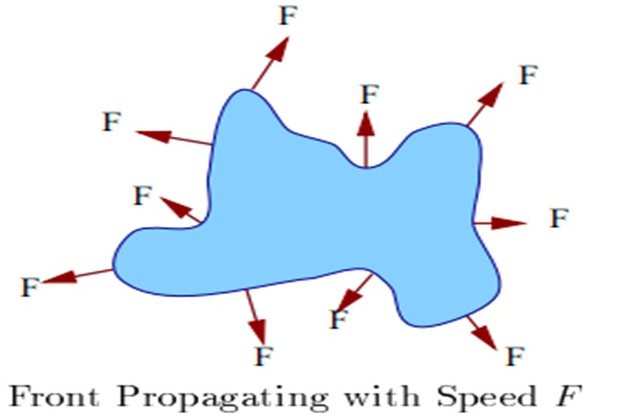描述曲线几何特征的两个重要参数是单位法矢和曲率，单位法矢描述曲线的方向，曲率则表述曲线弯曲的程度。曲线演化理论就是仅利用曲线的单位法矢和曲率等几何参数来研究曲线随时间的变形。曲线的演变过程可以认为是表示曲线在作用力 F 的驱动下，朝法线方向 N 以速度 v 演化。而速度是有正负之分的，所以就有如果速度 v 的符号为负，表示活动轮廓演化过程是朝外部方向的，如为正，则表示朝内部方向演化，活动曲线是单方向演化的，不可能同时往两个方向演化。
所以曲线的演变过程，就是不同力在曲线上的作用过程，力也可以表达为能量。世界万物都趋向于能量最小而存在。因为此时它是最平衡的，消耗最小的（不知理解对不？）。那么在图像分割里面，我们目标是把目标的轮廓找到，那么在目标的轮廓这个地方，整个轮廓的能量是最小的，那么曲线在图像任何一个地方，都可以因为力朝着这个能量最小的轮廓演变，当演变到目标的轮廓的时候，因为能量最小，力平衡了，速度为0了，也就不动了，这时候目标就被我们分割出来了。
那现在关键就在于：1）这个轮廓我们怎么表示；2）这些力怎么构造，构造哪些力才可以让目标轮廓这个地方的能量最小？
这两个问题的描述和解决就衍生出了很多的基于主动轮廓线模型的分割方法。第一个问题的回答，就形成了两大流派：如果这个轮廓是参数表示的，那么就是参数活动轮廓模型（parametric active contour model），典型为snake模型，如果这个轮廓是几何表示的，那么就是几何活动轮廓模型（geometric active contour model），即水平集方法（Level Set），它是把二维的轮廓嵌入到三维的曲面的零水平面来表达的（可以理解为一座山峰的等高线，某个等高线把山峰切了，这个高度山峰的水平形状就出来了，也就是轮廓了），所以低维的演化曲线或曲面，表达为高维函数曲面的零水平集的间接表达形式（这个轮廓的变化，直观上我们就可以调整山峰的形状或者调整登高线的高度来得到）。
那对于第二个问题，是两大流派都遇到的问题，是他们都需要解决的最关键的问题。哪些力才可以达到分割的目标呢？这将在后面聊到。
二、Snakes模型

自1987年Kass提出Snakes模型以来，各种基于主动轮廓线的图像分割理解和识别方法如雨后春笋般蓬勃发展起来。Snakes模型的基本思想很简单，它以构成一定形状的一些控制点为模板（轮廓线），通过模板自身的弹性形变，与图像局部特征相匹配达到调和，即某种能量函数极小化，完成对图像的分割。再通过对模板的进一步分析而实现图像的理解和识别。
简单的来讲，SNAKE模型就是一条可变形的参数曲线及相应的能量函数，以最小化能量目标函数为目标，控制参数曲线变形，具有最小能量的闭合曲线就是目标轮廓。
构造Snakes模型的目的是为了调和上层知识和底层图像特征这一对矛盾。无论是亮度、梯度、角点、纹理还是光流，所有的图像特征都是局部的。所谓局部性就是指图像上某一点的特征只取决于这一点所在的邻域，而与物体的形状无关。但是人们对物体的认识主要是来自于其外形轮廓。如何将两者有效地融合在一起正是Snakes模型的长处。Snakes模型的轮廓线承载了上层知识，而轮廓线与图像的匹配又融合了底层特征。这两项分别表示为Snakes模型中能量函数的内部力和图像力。
模型的形变受到同时作用在模型上的许多不同的力所控制，每一种力所产生一部分能量，这部分能量表示为活动轮廓模型的能量函数的一个独立的能量项。
Snake模型首先需要在感兴趣区域的附近给出一条初始曲线，接下来最小化能量泛函，让曲线在图像中发生变形并不断逼近目标轮廓。
Kass等提出的原始Snakes模型由一组控制点：v(s)=[x(s), y(s)] s∈[0, 1] 组成，这些点首尾以直线相连构成轮廓线。其中x(s)和y(s)分别表示每个控制点在图像中的坐标位置。 s 是以傅立叶变换形式描述边界的自变量。在Snakes的控制点上定义能量函数（反映能量与轮廓之间的关系）：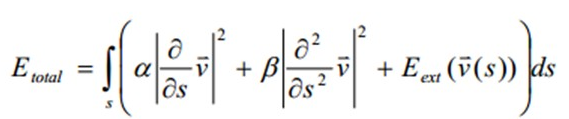其中第1项称为弹性能量是v的一阶导数的模，第2项称为弯曲能量，是v的二阶导数的模，第3项是外部能量（外部力），在基本Snakes模型中一般只取控制点或连线所在位置的图像局部特征例如梯度：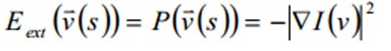也称图像力。（当轮廓C靠近目标图像边缘，那么C的灰度的梯度将会增大，那么上式的能量最小，由曲线演变公式知道该点的速度将变为0，也就是停止运动了。这样，C就停在图像的边缘位置了，也就完成了分割。那么这个的前提就是目标在图像中的边缘比较明显了，否则很容易就越过边缘了。）
弹性能量和弯曲能量合称内部能量（内部力），用于控制轮廓线的弹性形变，起到保持轮廓连续性和平滑性的作用。而第三项代表外部能量，也被称为图像能量，表示变形曲线与图像局部特征吻合的情况。内部能量仅仅跟snake的形状有关，而跟图像数据无关。而外部能量仅仅跟图像数据有关。在某一点的α和β的值决定曲线可以在这一点伸展和弯曲的程度。
最终对图像的分割转化为求解能量函数Etotal(v)极小化（最小化轮廓的能量）。在能量函数极小化过程中，弹性能量迅速把轮廓线压缩成一个光滑的圆，弯曲能量驱使轮廓线成为光滑曲线或直线，而图像力则使轮廓线向图像的高梯度位置靠拢。基本Snakes模型就是在这3个力的联合作用下工作的。
因为图像上的点都是离散的，所以我们用来优化能量函数的算法都必须在离散域里定义。所以求解能量函数Etotal(v)极小化是一个典型的变分问题（微分运算中，自变量一般是坐标等变量，因变量是函数；变分运算中，自变量是函数，因变量是函数的函数，即数学上所谓的泛函。对泛函求极值的问题，数学上称之为变分法）。
在离散化条件（数字图像）下，由欧拉方程可知最终问题的答案等价于求解一组差分方程：（欧拉方程是泛函极值条件的微分表达式，求解泛函的欧拉方程，即可得到使泛函取极值的驻函数，将变分问题转化为微分问题。）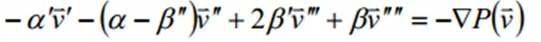记外部力 F = −∇ P， Kass等将上式离散化后，对x(s)和y(s)分别构造两个五对角阵的线性方程组，通过迭代计算进行求解。在实际应用中一般先在物体周围手动点出控制点作为Snakes模型的起始位置，然后对能量函数迭代求解。

Reference：

李天庆等，Snake模型综述，计算机工程，2005，第31卷 第9期

代码实现
% =========================================================================
%                   Snakes：Active Contour Models
% =========================================================================
% By gujinjin 2012/12/10-12  Sunny
% 基于KASS等的论文思想
% 参考文献：
%  KASS etc.Snakes：Active Contour Models
%  CSDN 博客 - Author：乐不思蜀Tone
%  Ritwik Kumar(Harvard University),D.Kroon（Twente University)的程序
%  《数学建模与数学实验》
% ------
clc;clf;clear all;

% =========================================================================
%                      获取手动取点坐标
% =========================================================================
% 读取显示图像
%I = imread('Coronary_MPR.jpg');
I =  imread('test3.png');
% 转化为双精度型
%I = im2double(I);
% 若为彩色，转化为灰度
if(size(I,3)==3), I=rgb2gray(I); end
figure(1),imshow(I);
%---------------------------
%        高斯滤波
%---------------------------
sigma=1.0;
% 创建特定形式的二维高斯滤波器H
H = fspecial('gaussian',ceil(3*sigma), sigma);
% 对图像进行高斯滤波,返回和I等大小矩阵
Igs = filter2(H,I,'same');
%---------------------------
%      获取Snake的点坐标
%---------------------------
figure(2),imshow(Igs);
x=[];y=[];c=1;N=100; %定义取点个数c,上限N
% 获取User手动取点的坐标
% [x,y]=getpts
while c<N
[xi,yi,button]=ginput(1);
% 获取坐标向量
x=[x xi];
y=[y yi];
hold on
% text(xi,yi,'o','FontSize',10,'Color','red');
plot(xi,yi,'ro');
% 若为右击，则停止循环
if(button==3), break; end
c=c+1;
end

% 将第一个点复制到矩阵最后，构成Snake环
xy = [x;y];
c=c+1;
xy(:,c)=xy(:,1);
% 样条曲线差值
t=1:c;
ts = 1:0.1:c;
xys = spline(t,xy,ts);
xs = xys(1,:);
ys = xys(2,:);
% 样条差值效果
hold on
temp=plot(x(1),y(1),'ro',xs,ys,'b.');
legend(temp,'原点','插值点');

% =========================================================================
%                     Snakes算法实现部分
% =========================================================================
NIter =1000; % 迭代次数
alpha=0.2; beta=0.2; gamma = 1; kappa = 0.1;
wl = 0; we=0.4; wt=0;
[row col] = size(Igs);

% 图像力-线函数
Eline = Igs;
% 图像力-边函数
[gx,gy]=gradient(Igs);
Eedge = -1*sqrt((gx.*gx+gy.*gy));
% 图像力-终点函数
% 卷积是为了求解偏导数，而离散点的偏导即差分求解
m1 = [-1 1];
m2 = [-1;1];
m3 = [1 -2 1];
m4 = [1;-2;1];
m5 = [1 -1;-1 1];
cx = conv2(Igs,m1,'same');
cy = conv2(Igs,m2,'same');
cxx = conv2(Igs,m3,'same');
cyy = conv2(Igs,m4,'same');
cxy = conv2(Igs,m5,'same');

for i = 1:row
for j= 1:col
Eterm(i,j) = (cyy(i,j)*cx(i,j)*cx(i,j) -2 *cxy(i,j)*cx(i,j)*cy(i,j) + cxx(i,j)*cy(i,j)*cy(i,j))/((1+cx(i,j)*cx(i,j) + cy(i,j)*cy(i,j))^1.5);
end
end

%figure(3),imshow(Eterm);
%figure(4),imshow(abs(Eedge));
% 外部力 Eext = Eimage + Econ
Eext = wl*Eline + we*Eedge + wt*Eterm;
% 计算梯度
[fx,fy]=gradient(Eext);

xs=xs';
ys=ys';
[m n] = size(xs);
[mm nn] = size(fx);

% 计算五对角状矩阵
% 附录: 公式（14） b(i)表示vi系数(i=i-2 到 i+2)
b(1)=beta;
b(2)=-(alpha + 4*beta);
b(3)=(2*alpha + 6 *beta);
b(4)=b(2);
b(5)=b(1);

A=b(1)*circshift(eye(m),2);
A=A+b(2)*circshift(eye(m),1);
A=A+b(3)*circshift(eye(m),0);
A=A+b(4)*circshift(eye(m),-1);
A=A+b(5)*circshift(eye(m),-2);

% 计算矩阵的逆
[L U] = lu(A + gamma.* eye(m));
Ainv = inv(U) * inv(L);

% =========================================================================
%                      画图部分
% =========================================================================
%text(10,10,'+','FontName','Time','FontSize',20,'Color','red');
% 迭代计算
figure(3)
for i=1:NIter;
ssx = gamma*xs - kappa*interp2(fx,xs,ys);
ssy = gamma*ys - kappa*interp2(fy,xs,ys);

% 计算snake的新位置
xs = Ainv * ssx;
ys = Ainv * ssy;

% 显示snake的新位置
imshow(I);
hold on;
plot([xs; xs(1)], [ys; ys(1)], 'r-');
hold off;
pause(0.001)
end  
运行效果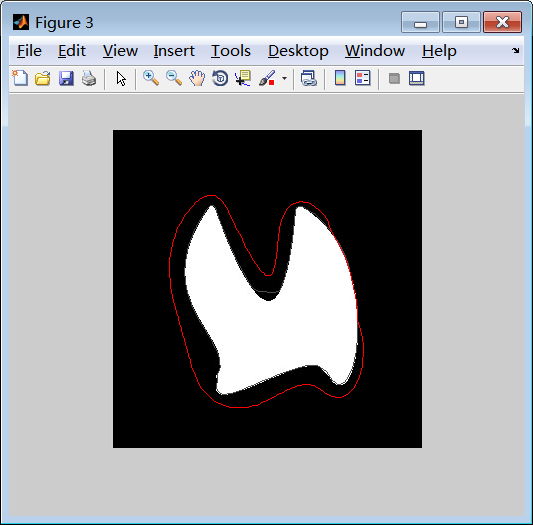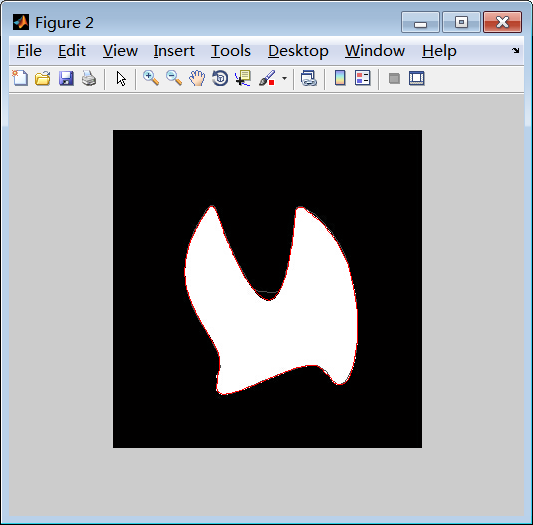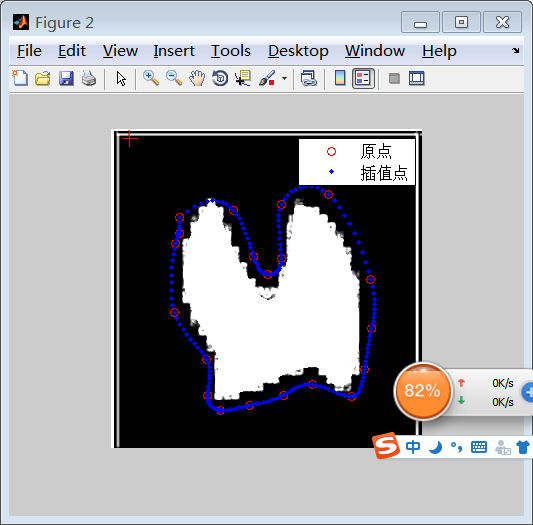展开全文• 它给出一个初始的轮廓，按照算法随着时间的推移，会最终收敛到物体的边缘，理论上来说包括： （1）几何活动轮廓，水平算法level-set algorithm（osher 1988） 理论上是给出一个初始曲线，随着时间推进，曲线总是在...
• matlab开发-使用明渠和活动传感器进行眼盘分割。该程序试图利用亮度通道和活动轮廓分割眼盘区域。
• 本人把CV, RSF and DRLSE等经典的活动轮廓模型转化成了基于Python3.6和Opencv3版本的代码，仅供参考。代码的运行效率比matlab快一个数量级。CV模型 图像分割 opencv
• 目前网上能找到的活动轮廓代码大都是matlab版本的，这里给出一个基于Python3.6和Opencv3版本的代码，仅供参考。注，代码的运行效率并不高，感觉没matlab快。 源代码下载地址： 写给出CV模型： #coding:utf-8 # ...Opencv3 python CV模型
• 关于活动轮廓模型（Active Contour Model）和水平集方法（Level Set Method），不多介绍了，是一种热门的图像分割算法，网上找到的代码基本上都是基于matlab的，运行效率很低。我用Opencv对一些比较有名的A...水平集方法 Opencv3
• % This Matlab program inplements geodesic active contour model. % Copyright (c) 2004--2007 by Chunming Li % Author: Chunming Li % email: li_chunming@hotmail.com 感谢原作者的大功无私，这里我把他的信息...
• 快速全局最小化活动轮廓图像分割源代码。 Xavier Bresson - April 10 2009 A Fast Global Minimization Algorithm for Active Contour Models Read: A Short Guide on a Fast Global Minimization Algorithm for ...
• 一、粒子群算法理论粒子群算法来源于鸟类集体活动的规律性，进而利用群体智能建立简化模型。它模拟的是鸟类的觅食行为，将求解问题的空间比作鸟类飞行的时间，每只鸟抽象成没有体积和质量的粒子，来表征一个问题的...
• 活动轮廓模型;matlab 编程 1 模型的建立 在图像中, 对象与背景的区别有时表现为平均灰度的明显不同. 由于这类图像既没有明显的边缘 ($\sev{\n I}$ 大), 也缺乏明显的纹理 (texture, 灰度变化有一定的规律, 并...
• matlab开发-Activecontourplatform。比较不同层次集和活动轮廓法的性能。
• matlab开发-ActiveContourSegmentation。使用级别集和活动轮廓查找前景和背景区域。
• matlab开发-LocalizedActiveContour。此包使用级别集方法实现本地化的活动轮廓方法# matlab活动轮廓matlab 订阅